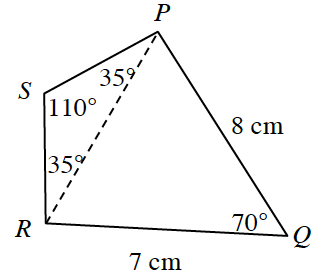### Home > CCG > Chapter 5 > Lesson 5.3.5 > Problem5-134

5-134.Use your triangle tools to solve the problems below.

1. Find $PR$  in the diagram at right.

Use the Law of Cosines to solve for the length of segment $PR$.
Relabel the parts of the triangle so they match the formula if you need more assistance.

Refer to the Math Notes box in Lesson 5.3.3 if you need help remembering what this is.

2. Find the perimeter of quadrilateral $PQRS$.

Use the Law of Sines to find the length of segment $PS$.
If you need more assistance refer to Math Note box in Lesson 5.3.1.

$\frac{\sin35º}{PS}=\frac{\sin110º}{PR}$

Keep in mind $RS=PS$, because $ΔRSP$ is an isosceles triangle, because the base angles are equal at $35º$ each.

$\text{perimeter}=25.55$ cm